C++ 迭代器，了解一下？

2018-08-11 17:32:56

迭代器是个啥？#include <iterator>

迭代器能干啥？

int main(){
/*
* suppose:
* T t;
* container<T> v1{t}, v2;
* container<T>::iterator
* container<T>::begin()
* container<T>::end()
* have been defined here
*/
container<T>::iterator ita = v1.begin(), itb = v2.begin();
*ita;
using std::swap;
swap(ita, itb);
++ita;
}

emmmm...这些跟指针没什么差别对吧。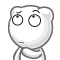真的没差别吗？

*it返回什么是未指定的，返回void也合fa♂ 三去法，意味着在这里你可能什么也做不了2333333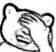那我咬着要这迭代器有何用？

template< class Iterator >
struct iterator_traits {
typedef typename Iterator::difference_type difference_type;
typedef typename Iterator::value_type value_type;
typedef typename Iterator::pointer pointer;
typedef typename Iterator::reference reference;
typedef typename Iterator::iterator_category iterator_category;
};

struct output_iterator_tag { };
struct input_iterator_tag { };
struct forward_iterator_tag : public input_iterator_tag { };
struct bidirectional_iterator_tag : public forward_iterator_tag { };
struct random_access_iterator_tag : public bidirectional_iterator_tag { };

迭代器分类

• 输出迭代器(OutputIterator)
• 输入迭代器(InputIterator)
• 向前迭代器(ForwardIterator)
• 双向迭代器(BidirectionalIterator)
• 随机访问迭代器(RandomAccessIterator)
• 相接迭代器(ContiguousIterator)

• 可变迭代器(MutableIterator)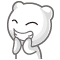由上至下越来越像指针，越来越正常

输出迭代器

typedef output_iterator_tag iterator_category;

仅资瓷单趟算法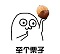输出迭代器这个名字看起来就是用于输出内容的，STL有两个小玩意叫做std::ostream_iteratorstd::ostreambuf_iterator，把输出流包装了一下

// 构造一个输出T类型的输出迭代器
// 第一个参数为绑定到的流
// 第二个参数为每次输出后输出的字符串，可省
std::ostream_iterator<T> it(std::cout, " ");
T t;
it = t; //这些都等同于 std::cout << t << " "
*it = t;
it++ = t;
++it = t;
*it++ = t;

// 跟上面那个一样，不过变成输出字符类型，也没有第二个参数了
std::ostreambuf_iterator<char> it(std::cout);

it = 'A'; // 等同于 std::cout << 'A'
*it = 'A';
...

STL还有几个用于插入序列的迭代器适配器，以及配套的为了方便的函数模板

std::deque<int> q;

std::back_insert_iterator< std::deque<int> > it1(q);
// 等同于 auto it1 = std::back_inserter(q);
it1 = 1; // 等同于 q.push_back(1)
*it1 = 1;
...

std::front_insert_iterator< std::deque<int> > it2(q);
// 等同于 auto it2 = std::front_inserter(q);
it2 = 1; // 等同于 q.push_front(1)
*it2 = 1;
...

std::insert_iterator< std::deque<int> > it3(q, q.begin());
// 等同于 auto it3 = std::inserter(q);
it3 = 1; // 等同于 q.insert(q.begin(), 1)
*it3 = 1;
...

输入迭代器

typedef input_iterator_tag iterator_category;

operator*返回reference，为可转换为value_type的引用std::vector<bool>为了节约空间，每一个 $01$ 位占一个bit而非一个byte，但是没有办法返回一个bit的对象，只能返回一个包装好的代理类辣，所以std::vector<bool>::iterator::reference是代理类的引用而非位引用或bool&

PS:std::vector<bool>不是容器，不满足容器的要求，下文提到std::vector时均不包含std::vector<bool>

C++真是一门难学的语言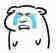仅资瓷单趟算法把输出迭代器的栗子的输出改成输入就好了

emmmmm...等等，那EOF咋判断？std::vector<int> v;
std::istream_iterator<int> i1(std::cin), i2;
while(i1 != i2) v.push_back(*i1++);

std::istream_iterator<int> i1(std::cin);
int a = *i1, b = *i1, c = *++i1, d = *i1++; // 前提未EOF

std::istreambuf_iterator同理，只不过读入的变成了字符那这个输入/输出迭代器比直接用std::cin/cout还麻烦啊！！！有啥用啊！！！众所周知，std::vector有这样一个构造函数，接收一对迭代器，将 $[begin,end)$ 范围内元素拷贝入容器（std::vector<>::assign()也是）

std::vector<int> v{std::istream_iterator<int>(std::cin), std::istream_iterator<int>()};

向前迭代器

typedef forward_iterator_tag iterator_category;
提供多趟保证

• 要么二者都不可解引用，要么指向同一对象
• ++ita == ++itbstd::forward_list和无序关联容器（哈希容器）的iterator

双向迭代器

typedef bidirectional_iterator_tag iterator_category;
可自减std::list和有序关联容器的iterator

随机访问迭代器

typedef random_access_iterator_tag iterator_category;
可以使用operator[]std::vector, std::array, std::deque, std::stringiterator、指向数组元素的指针

相接迭代器

其所指向的逻辑相邻元素也在内存中物理上相邻C++真是一门难学的语言std::vector, std::array, std::basic_string_viewiterator、指向数组元素的指针

可变迭代器

是输出迭代器所有STL容器的iteratorconst_iterator除外）、指针（常量指针除外）

类型总结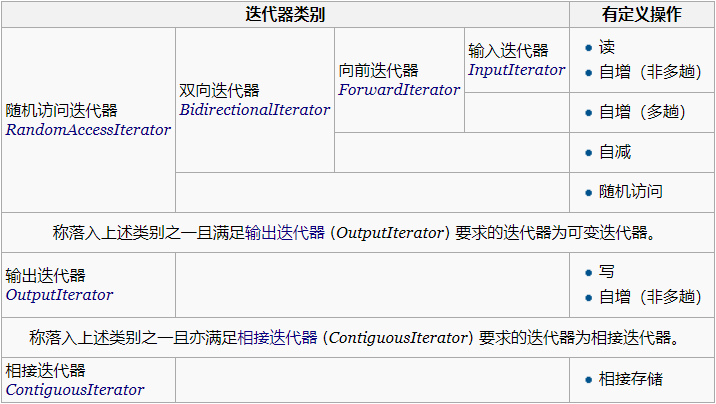相对用的比较多的迭代器适配器

std::reverse_iteratorSTL容器的rbegin(), rend()返回的就是这个

迭代器非法化

（溜了溜了）

emmmm...还是说几个常见的吧

std::vector扩大重分配、std::deque插入+扩大重分配+非首尾擦除：全部

std::vector插入/擦除（无重分配）：插入位置及其后

std::deque首尾擦除（无重分配）：首尾

（毕竟它们是节点形式出现的）

一点废话但是对于非内置类型就不一定了

参考资料

（德）约祖蒂斯（Josuttis,N.M.）著；侯捷译.C++标准库：第2版[M].北京：电子工业出版社，2015.6：433-474.

• star
首页